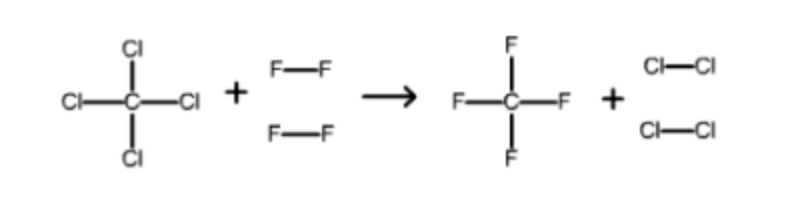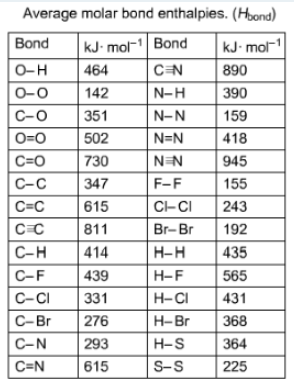# Problem: Use the molar bond enthalpy data in the table to estimate the value of ΔH°rxn for the equationCCI4(g) + 2F2(g) → CF4(g) + 2CI2(g

###### FREE Expert Solution
85% (216 ratings)
###### FREE Expert Solution

To find the enthalpy of formation in terms of bond energies, use the equation:

ΔHrxn = {Bond energy of reactants} - {Bond energy of products}-The first reactant has 4 C-Cl bonds. Each C-Cl bond has a bond enthalpy of 331 kJ/mol.

331 x 4 = 1324 kJ/mol

85% (216 ratings)###### Problem Details

Use the molar bond enthalpy data in the table to estimate the value of ΔH°rxn for the equation

CCI4(g) + 2F2(g) → CF4(g) + 2CI2(gWhat scientific concept do you need to know in order to solve this problem?

Our tutors have indicated that to solve this problem you will need to apply the Bond Energy concept. You can view video lessons to learn Bond Energy. Or if you need more Bond Energy practice, you can also practice Bond Energy practice problems.

What is the difficulty of this problem?

Our tutors rated the difficulty ofUse the molar bond enthalpy data in the table to estimate th...as medium difficulty.

How long does this problem take to solve?

Our expert Chemistry tutor, Sabrina took 8 minutes and 27 seconds to solve this problem. You can follow their steps in the video explanation above.

What professor is this problem relevant for?

Based on our data, we think this problem is relevant for Professor Ardo's class at UCI.